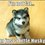I have come across this type of problems many times in brilliant(sure you would to)

A monic polynomial of degree $N$ leaves the remainder:-

$A$ when divided by $(x \pm n_{1})$

$B$ when divided by $(x \pm n_{2})$

$C$ when divided by $(x \pm n_{3})$

$D$ when divided by $(x \pm n_{4})$

$E$ when divided by $(x \pm n_{5})$

$F$ when divided by $(x \pm n_{6})$

and so on.....

Find out the value of $P(Z)$

So, I just wanted to know a more or less shortcut method to solve this type of problem rather than just to plug the values and solve the equations(this can sometimes become a hilarious job)..

Any type of help will be appreciated.

$\color{limegreen}{\text{Thank you}}$.Note by Anik Mandal
6 years, 6 months ago

This discussion board is a place to discuss our Daily Challenges and the math and science related to those challenges. Explanations are more than just a solution — they should explain the steps and thinking strategies that you used to obtain the solution. Comments should further the discussion of math and science.

When posting on Brilliant:

• Use the emojis to react to an explanation, whether you're congratulating a job well done , or just really confused .
• Ask specific questions about the challenge or the steps in somebody's explanation. Well-posed questions can add a lot to the discussion, but posting "I don't understand!" doesn't help anyone.
• Try to contribute something new to the discussion, whether it is an extension, generalization or other idea related to the challenge.

MarkdownAppears as
*italics* or _italics_ italics
**bold** or __bold__ bold
- bulleted- list
• bulleted
• list
1. numbered2. list
1. numbered
2. list
Note: you must add a full line of space before and after lists for them to show up correctly
paragraph 1paragraph 2

paragraph 1

paragraph 2

[example link](https://brilliant.org)example link
> This is a quote
This is a quote
    # I indented these lines
# 4 spaces, and now they show
# up as a code block.

print "hello world"
# I indented these lines
# 4 spaces, and now they show
# up as a code block.

print "hello world"
MathAppears as
Remember to wrap math in $$ ... $$ or $ ... $ to ensure proper formatting.
2 \times 3 $2 \times 3$
2^{34} $2^{34}$
a_{i-1} $a_{i-1}$
\frac{2}{3} $\frac{2}{3}$
\sqrt{2} $\sqrt{2}$
\sum_{i=1}^3 $\sum_{i=1}^3$
\sin \theta $\sin \theta$
\boxed{123} $\boxed{123}$

Sort by:

@Aditya Raut @Satvik Golechha @Calvin Lin @Krishna Ar @Sandeep Bhardwaj @Trevor Arashiro @Finn Hulse @Agnishom Chattopadhyay @brian charlesworth and all other $\color{#D61F06}{\text{Brilliant' ians}}$

- 6 years, 6 months ago

Yes. Satvik is right. Method of differences is easy to employ too.

- 6 years, 6 months ago

If all the $n$'s are consecutive, you can use the Method of Differences. Check it's Wiki.

- 6 years, 6 months ago

Thanks...

- 6 years, 6 months ago

Anik, because the polynomial problems are linear, we can always solve it using matrix. For example for the problem: Am I cubic?, my solution is as follows. I use an Microsoft Excel spreadsheet to do the matrix calculations.

Assuming that $f(x)$ is a cubic polynomial, we can write in matrix form:

$XA=B\quad \Rightarrow \begin{bmatrix} 1^3 & 1^2 & 1^1 & 1^0 \\ 2^3 & 2^2 & 2^1 & 2^0 \\ 3^3 & 3^2 & 3^1 & 3^0 \\ 4^3 & 4^2 & 4^1 & 4^0 \end{bmatrix} \begin{bmatrix} a_3 \\ a_2 \\ a_1 \\ a_0 \end{bmatrix} = \begin{bmatrix} 4 \\ 3 \\ 4 \\ 7 \end{bmatrix}$

We can find $A$ as follows:

$A = X^{-1}B = \begin{bmatrix} -\frac{1}{6} & \frac {1}{2} &-\frac {1}{2} & \frac {1}{6} \\ \frac {3}{2} & -4 & \frac {7}{2} &-1 \\ -\frac {13}{3} & \frac {21}{2} & -7 & \frac{11}{6} \\ 4 & -6 & 4 & -1 \end{bmatrix} \begin{bmatrix} 4 \\ 3 \\ 4 \\ 7 \end{bmatrix} = \begin{bmatrix} 0 \\ 1 \\ -4 \\ 7 \end{bmatrix}$

It is shown that: $f(x) = x^2-4x+7$ showing that: $\boxed{No}$, it is not a cubic polynomial.- 6 years, 6 months ago

Thanks@Chew-Seong Cheong

- 6 years, 6 months ago

Sir how did we conclude that it is quadratic polynomial

- 6 years, 5 months ago

Gaurav, $A = \{0, 1, -4, 7\}$ means that $f(x) = (0)x^3 + x^2-4x+7$.

- 6 years, 5 months ago

Oh! I got , Thank you Sir

- 6 years, 4 months ago